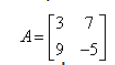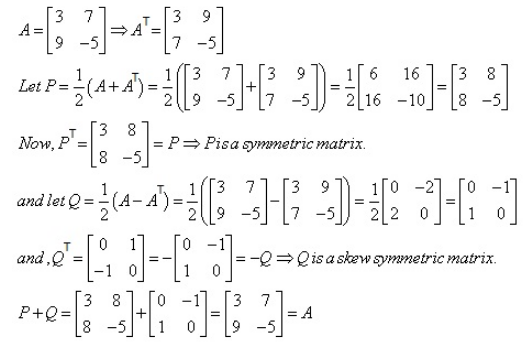# Express the matrix as the sum of a symmetric and a skew-symmetric matrix

Express the matrixas the sum of a symmetric and a skew-symmetric matrix.